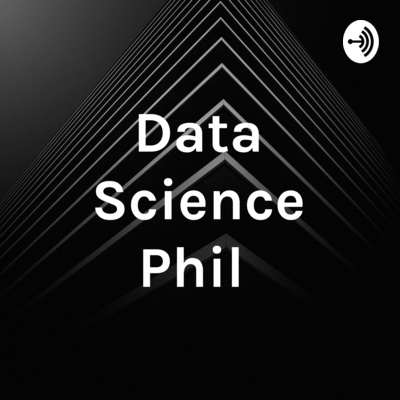# Data Science Phil

By Philipp Packmohr
In this podcast we talk about data science and related topics
##### Where to listen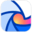Breaker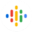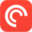Pocket Casts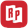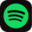Spotify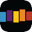StitcherThe Fermi Problem
In this episode I talk to Bence Mélykúti, DPhil.  Bence has an MSc degree (incl. undergrad studies) in mathematics from the Mathematical Institute of the Eötvös Loránd University (ELTE), Budapest, Hungary. He defended his doctoral thesis in January 2011 at the University of Oxford, UK, where he was with the Department of Statistics and the Life Sciences Interface Doctoral Training Centre as a member of Keble College. He worked under the supervision of Prof. Alison Etheridge (Dept. of Statistics) and Dr. Antonis Papachristodoulou (Control Group, Dept. of Engineering Science). His Research interests include: The interfaces of mathematical (esp. probabilistic) modelling,  stochastic processes, statistics, machine learning, chemical reaction  kinetics, systems biology, control theory, operations research. He seeks novel possibilities how these can be applied to develop quantitative methods for research in the life sciences. He is also interested in making  a positive societal impact with his research, and is open to being  contacted with interesting project ideas for consultation.  Further Bio: He was based at the Centre for Biological Systems Analysis (ZBSA) and tangentially at the Department of Mathematical Stochastics, both at the University of Freiburg, Germany. His host there was Prof. Peter Pfaffelhuber. In Freiburg, he was most recently an AXA Research Fund postdoctoral fellow. He was visiting the Isaac Newton Institute for Mathematical Sciences, Cambridge, UK, from January until June 2016.  He came to Freiburg with a Humboldt Postdoctoral Research Fellowship. Before that, he was a postdoctoral researcher at the Department of Mechanical Engineering, University of California, Santa Barbara. He worked with Profs. Mustafa Khammash (Dept. of Mechanical Engineering) and João P. Hespanha (Dept. of Electrical and Computer Engineering). In this episode we talk about the German Tank Problem, the Mark and Recapture Problem and the Fermi problem. Bence recommnded the Quanta magazine.
1:26:30
September 24, 2019The case-crossover design via penalized regression
In this episode I talk to Sam Doerken.  Sam is a mathematician by training, having studied mathematics at the  University of Heidelberg. He did his diploma thesis in mathematics on Probabilistic Forecasting of U.S. Treasury Bills . Since 2012 he works at the  Institute of Medical Biometry and Statistics at the University of Freiburg. In this part we cover the paper "The case-crossover design via penalized regression" , published in BMC Medical Research Methodology. The authors conclude that "for the case-crossover design, we also encourage penalized regression for routine use."
13:10
September 17, 2019Probabilistic Forecasting of U.S. Treasury Bills
In this episode I talk to Sam Doerken. Sam is a mathematician by training, having studied mathematics at the University of Heidelberg. He did his diploma thesis in mathematics on Probabilistic Forecasting of U.S. Treasury Bills . Since 2012 he works at the Institute of Medical Biometry and Statistics at the University of Freiburg.  In this episode we talk abot the topic of his diploma thesis Probabilistic Forecasting of U.S. Treasury Bills and we cover time series analysis.
14:59
September 10, 2019Theory of Distributed Computation
In this episode I talk to Philipp Schneider, PhD student in theoretical computer science in Fabian Kuhns group at the University of Freiburg. Before that he studied computer science at the Karlsruhe Institute of Technology. Philipps reseach is concerned with the Theory of Distributed Computation. In distributed computation one assumes processors (or computation nodes) that are far away from each other. The input of some problem is distributed on these computing nodes and they have to collaborate to compute the solutions. The goal is to optimize the rescources, which in this case is communication, specifically the number of communication rounds. The goal of the research is twofold: (1) Upper bounds: Designing algorithms that solve a given problem in a given distributed computational model with as little communication as possible. (2) Lower bounds: Showing the intrinsic "hardness" of certain problems in a given computational model, by proving that they require a certain minimum amount of communication. A typical problem in this regard is graph coloring, where the nodes of a graph must be colored with as few colors as possible, such that all neighboring nodes have different colors. We discussed the seminal paper "LOCALITY IN DISTRIBUTED GRAPH ALGORITHMS" by NATHAN LINIAL which considers a simple distributed computational model called the LOCAL model where the communication network is a graph and nodes are processessing units that act synchronously in rounds. Nodes can send a messages to each neighbors in the graph in each round. The problem is to color this communication graph. The paper uses a mathematical structure called cover free sets by the mathematician Erdös. Given a graph which is already correctly colored with k colors, Linial uses this concept to push the number of colors down to roughly log(k) (with some simplifying lies) in a single round of communication. This step can be repeated several times (until the number of colors gets small and other factors play a role), and leads to a fast solution for coloring with few colors. This gives an "upper bound" for the communication required to solve the graph coloring problem. Linial complements this result by showing that this number of rounds is actually required to color a worst case graph with a number of colors that is close to the theoretical minimum, i.e. he gives a "lower bound". We also talked about the Massively Parallel Computation model (MPC model): The MPC model is used in Big Data settings. The huge input is distributed over several machines. The machines have restricted memory and can send and receive at most (roughly) size of memory bits per round in an all-to-all communcation network. This captures the characteristics of distributed data centers and can be considered as theoretical counterpart to the rather famous MapReduce programming model of Google. In the end we talk about the importance of a general computer science curriculum in school education. Philipp recommended "The economist" as a good journalistic publication.
41:10
August 26, 2019Open problems in mathematics
In this episode I talk to Gaetan Leclerc about some open problems in mathematics, part of the so called Millenium problems. Gaeton is a first year master student at the ENS Rennes. Before that he studied mathematics at the École Normale Supérieure de Rennes (ENS Rennes).  We cover the Navier-Stokes equations which are very important in computational fluid dynamics and thus have many applications in engineering, medicine, biology and climate science. The mathematician Grigori Perelman presented a proof of the Poincaré conjecture in three papers made available in 2002 and 2003 on the arXiv. We also talk about the P vs NP problem in computer science. Gaetan recommended the math YouTuber 3Blue1Brown for his great visualizations of complex topics.
32:19
August 10, 2019